# Ion Selective Electrode Measurement - Online ISE Analysis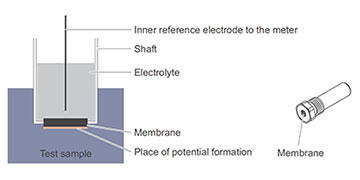## Online Ion Selective Electrode Analysis

There are various analytical measuring techniques to determine the concentrations of substances in unknown samples, e.g. photometry, titration, or mass spectrometry. One advantage of working with ion sensitive electrodes (ISE), is the possibility of obtaining data using an economical alternative vs otherwise potentially expensive instrumentation.

ISEs are electrochemical sensors that can be used directly in the mg/L range. YSI advancements in ISE measurements demonstrate that this principle is also suitable for continuous online operations.

ISE analysis is a potentiometric procedure. This means the desired signal occurs in the form of a potential, more precisely of potential differences, or voltages in mV.

An ion sensitive measuring system basically consists of the ISE reacting on a special ion type and a reference electrode that is jointly immersed in the sample to be measured (see figure below).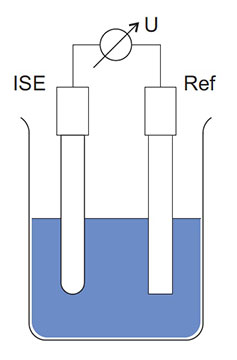Schematic structure of an ion sensitive measuring system with ISE (ion sensitive electrode),
Ref (reference electrode) and voltmeter.

This combination is called a measuring chain. This type of instrument must be highly resistive in order to fulfill the conditions of a potentiometric measurement. Only then are the measurement currents low enough so no value-changing polarization occurs and the electrodes are not damaged. For these reasons, the electrode connections must be kept dry. Moisture can cause unwanted parallel connections and interferences.

### The Nernst Equation

The ion sensitive electrode provides an electrochemical potential that is influenced by the concentration of the ion type to be measured. The reference electrode, however, is intended to build up an electrochemical potential that does not depend on the composition of the sample to be measured. The difference of these potentials, the voltage displayed by the meter, can be described by the Nernst equation:U0 ion is a fixed value given by the measuring system. The slope S defines how much the measurement signal is increased or reduced when the concentration changes. Its theoretical value is referred to as the Nernst slope and is 59.16 mV at 25°C for simply charged ions such as ammonium or nitrate. The arithmetic sign depends on the charge of the measured ions, a plus is for positively charged cations, e.g. K+, a minus for anions such as Cl-.

The activity a is the active concentration of an ion type that takes into account the influence of other ions in the sample and thus describes a considerable part of the matrix effect. Suitable procedures for calibrating and measuring make it possible to work in practice with the adapted form of the Nernst equation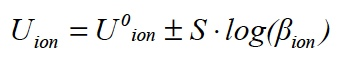where β is the mass concentration, for example, mg/L.

As an example, a graphic of this function is shown in Fig 2. Note that the concentration value is scaled logarithmically. The measured voltages are mostly in the range -500 mV to +500 mV.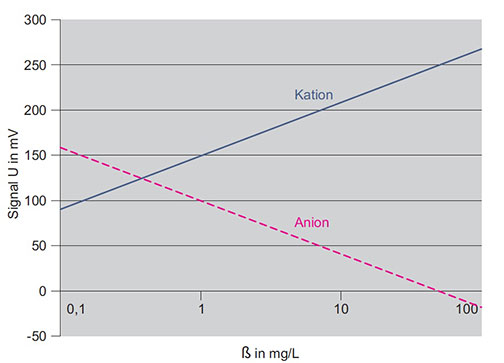Theoretical calibration lines for anions and cations (according to Nernst equation)

### ISE Electrodes - Structure and Function

An ISE has a shaft with a built-in membrane (see figure below). The membrane is the essential sensing device and guarantees the sensitivity for a certain ion type. The ion specific potential develops on the membrane surface according to the Nernst equation.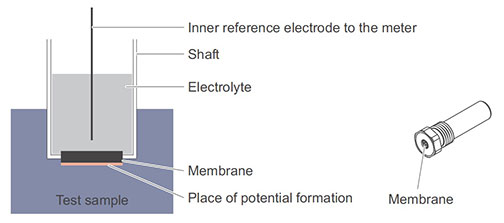Structure of an ion sensitive electrode (diagram on the left, sample model on the right)

There are various types of membranes. The most important types are glass membranes, solid body membranes, and synthetic material membranes.

#### Glass Membranes

The ion selective membrane most frequently used is the glass membrane, which is primarily used in pH measurements. The pH sensitivity is due to a thin expanding layer in the glass through which ions can diffuse in and out. Other ions, e.g. Na+, can also be determined with the corresponding glass types and special procedures.

#### Solid Body Membranes

Membranes made out of hardly soluble salts such as lanthanum fluoride, silver chloride, or silver sulfide can also be used to detect e.g. fluoride, chloride, sulfide, or silver.

#### Synthetic Material Membranes

Certain synthetic materials are able to absorb ion sensitive substances. Thus, after the addition of so-called ionophores, PVC that contains plasticizers is suitable for measuring various ions.

Contrary to the theoretical graphs, curves in practice are often like those in the figure below.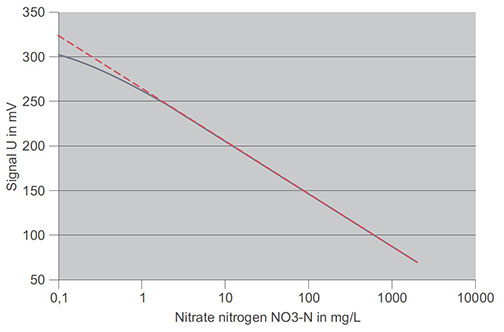Real characteristic curve of a nitrate ISE (solid line) and purely theoretical Nernst function (dashed)

This real characteristic curve differs from the straight line function in the concentration range below 1 mg/L nitrate-N. Here, the electrode reacts less strongly to the required measured parameter.

In YSI measuring systems this effect is taken into account automatically. If the concentrations are even lower, the value is so low the ISE no longer reacts to changes of the measured ion. This value is referred to as the detection limit and is different for individual electrode types. It also depends on the presence of other interfering ions.

For the tasks required in wastewater treatment plants, electrodes for the parameters ammonium and nitrate are useful. In addition, potassium and chloride electrodes can be used for compensation purposes. The application range of the first two parameters can thus be extended.

# Additional Blog Posts of Interest:

ORP Management in Wastewater as an Indicator of Process Efficiency

What's Next for Water Quality Sensor Trends in Wastewater?

Myths and Realities: Ammonium Based Aeration Control in Wastewater

Solving Water Resource Recovery Issues with Monitoring and Control